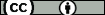## Optimal Gersgorin-style estimation of the largest singular value. II

Johnson, C.R. ; Peña, J.M. (Universidad de Zaragoza) ; Szulc, T.
Resumen: In estimating the largest singular value in the class of matrices equiradial with a given $n$-by-$n$ complex matrix $A$, it was proved that it is attained at one of $n(n-1)$ sparse nonnegative matrices (see C.R.~Johnson, J.M.~Pe{\~n}a and T.~Szulc, Optimal Gersgorin-style estimation of the largest singular value; {\em Electronic Journal of Linear Algebra Algebra Appl.}, 25:48--59, 2011). Next, some circumstances were identified under which the set of possible optimizers of the largest singular value can be further narrowed (see C.R.~Johnson, T.~Szulc and D.~Wojtera-Tyrakowska, Optimal Gersgorin-style estimation of the largest singular value, {\it Electronic Journal of Linear Algebra Algebra Appl.}, 25:48--59, 2011). Here the cardinality of the mentioned set for $n$-by-$n$ matrices is further reduced. It is shown that the largest singular value, in the class of matrices equiradial with a given $n$-by-$n$ complex matrix, is attained at one of $n(n-1)/2$ sparse nonnegative matrices. Finally, an inequality between the spectral radius of a $3$-by-$3$ nonnegative matrix $X$ and the spectral radius of a modification of $X$ is also proposed.
Idioma: Inglés
DOI: 10.13001/1081-3810.3033
Año: 2016
Publicado en: Electronic Journal of Linear Algebra 31 (2016), 679-685
ISSN: 1537-9582

Factor impacto JCR: 0.475 (2016)
Categ. JCR: MATHEMATICS rank: 222 / 310 = 0.716 (2016) - Q3 - T3
Factor impacto SCIMAGO: 0.604 - Algebra and Number Theory (Q3)

Financiación: info:eu-repo/grantAgreement/ES/DGA/FSE
Financiación: info:eu-repo/grantAgreement/ES/MINECO-FEDER/MTM2015-65433-P
Tipo y forma: Article (Published version)You must give appropriate credit, provide a link to the license, and indicate if changes were made. You may do so in any reasonable manner, but not in any way that suggests the licensor endorses you or your use.

Este artículo se encuentra en las siguientes colecciones:
Articles